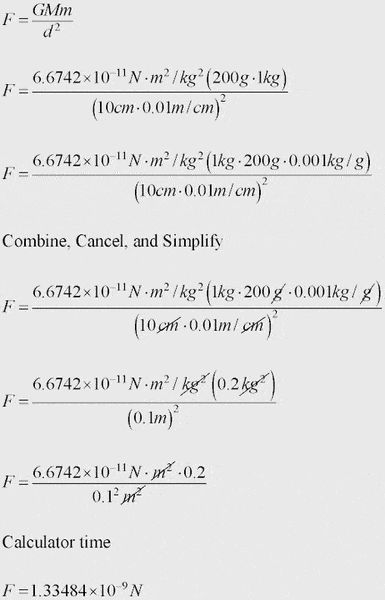# Solving an Equation using the Gravitational Constant

Thrawn

## Homework Statement

Why, in the second step of the question, is 0.001 added to the right side?

Part 2. In the equation Ve = sqrt 2(6.6742 x 10^-11 N m^2 / kg^2 (12.1kg))/0.106780959m

I'm trying to calculate the escape velocity of an object, and have figured out everything up to this point. Now, I have no idea how to procede. Do I cancel things out? Do I add a number to both sides?

## Homework Equations## The Attempt at a Solution

For the first part, I would guess that it is simply to... well I have no idea...

Staff Emeritus

## Homework Statement

Why, in the second step of the question, is 0.001 added to the right side?
200 is multiplied by 0.001 to convert it from grams into kilograms, since there are 1000g in 1kg-- does that answer your question?
Part 2. In the equation Ve = sqrt 2(6.6742 x 10^-11 N m^2 / kg^2 (12.1kg))/0.106780959m

I'm trying to calculate the escape velocity of an object, and have figured out everything up to this point. Now, I have no idea how to procede. Do I cancel things out? Do I add a number to both sides?

I don't get what you mean by this!

Thrawn
200 is multiplied by 0.001 to convert it from grams into kilograms, since there are 1000g in 1kg-- does that answer your question?

I don't get what you mean by this!

The formula to calculate escape velocity is:

Ve = sqrt 2(mu)/r

Where r is the distance from the denter of the object to be escaped from, and mu is the Gravitational Constant multiplied by the mass of the object to be escaped from.

All sai and done, this gives an equation of: Ve = sqrt 2(6.6742 x 10^-11 N m^2 / kg^2 (12.1kg))/0.106780959m

What would be the next step in solving this?

Staff Emeritus
You just need to check that the units cancel to give a unit of velocity (noting that N=kg m s-2), then compute the numerical answer.

Thrawn
You just need to check that the units cancel to give a unit of velocity (noting that N=kg m s-2), then compute the numerical answer.

So would the next step be: Ve = sqrt 2(6.6742 x 10^-11 kg m/s^2 m^2/kg^2 (12.1kg)/0.106780959m ?

If not, then what?

Staff Emeritus